# What is the best shape for a soccer goal post?

kshitij
square better than round for a shot from an acute angle.
and how can you say this?

As I mentioned in my post a square post has 33% chance of shot going in after hitting the post, whereas a round post has at-least 25% chance. How much more than 25% is what I wanted to calculate but the math is tricky for me.

Homework Helper
Gold Member
2022 Award
and how can you say this?

As I mentioned in my post a square post has 33% chance of shot going in after hitting the post, whereas a round post has at-least 25% chance. How much more than 25% is what I wanted to calculate but the math is tricky for me.
Suppose the ball approaches at an angle ##\theta## to the normal (so an agle of zero is normal to the goal line) and at a lateral offset from the centre of the post of x.
So we only need to consider x in the range -R to +R, the positive side being where the actual goal is.
E.g. with ##\theta=0##, it will go in the goal if ##x>R/\sqrt 2##, yes?
Can you generalise that?

Last edited:
Homework Helper
Gold Member
I only need a rough idea about which post is better.
The concept of a 'rough idea' is not meaningful here: either the square post is 'better', or the round post is, or they are 'equal'. The square post cannot be approximately better than the round post.

If say somehow we could conclude that the round post is better than the square one for a point sized ball
,
We would say that this is an (exact) solution to a simplified problem, rather than a 'rough idea' of the solution of the main problem.

then I would be happy to say that it should also be better for finite sized ball even though this might not be true
That may be enough for you, but it would not be a reasonable conclusion for a scientist to make.

The solution for a point-sized ball is trivial: any shot that strikes the inside surface of a square post will go in, whereas some shots that strike the inside/pitch-side quadrant of a round post will bounce out. A simple sketch confirms that any trajectory that strikes the  forward half of the [end edit] inside surface of a square post would strike a round post in that quadrant. The square post is 'better' (lets more goals in).

Last edited:
Homework Helper
Gold Member
My guess is that it depends on the angle of the shot: round may be better than square posts for a direct shot and square better than round for a shot from an acute angle.
I agree with that guess.

One proviso is the complexity of a collision between a ball and the corner of a square post - and the friction between the ball and post in general. It won't be an elastic collision.
Indeed, and again my guess is that in in the majority of the discriminating cases the ball will strike the corner of a square post so that for a meaningful answer you would have to take the complexities into account, and confirm any calculations by experiment.

kshitij
The solution for a point-sized ball is trivial: any shot that strikes the inside surface of a square post will go in, whereas some shots that strike the inside/pitch-side quadrant of a round post will bounce out. A simple sketch confirms that any trajectory that strikes the  forward half of the [end edit] inside surface of a square post would strike a round post in that quadrant. The square post is 'better' (lets more goals in).
I am not sure what your sketch is but if I understood you correctly then I think you meant something like if we choose a particular trajectory of ball then simultaneously compare what happens when this trajectory strikes the surface of the square & circle, then we will find that all the trajectories striking sector BC (see the figure in my original post) that will go in for the round post will also go in for the square post but some trajectories which won't go in for the circle will go in for the square

kshitij
I am not sure what your sketch is but if I understood you correctly then I think you meant something like if we choose a particular trajectory of ball then simultaneously compare what happens when this trajectory strikes the surface of the square & circle, then we will find that all the trajectories striking sector BC (see the figure in my original post) that will go in for the round post will also go in for the square post but some trajectories which won't go in for the circle will go in for the square
So, the sketch should look something like this?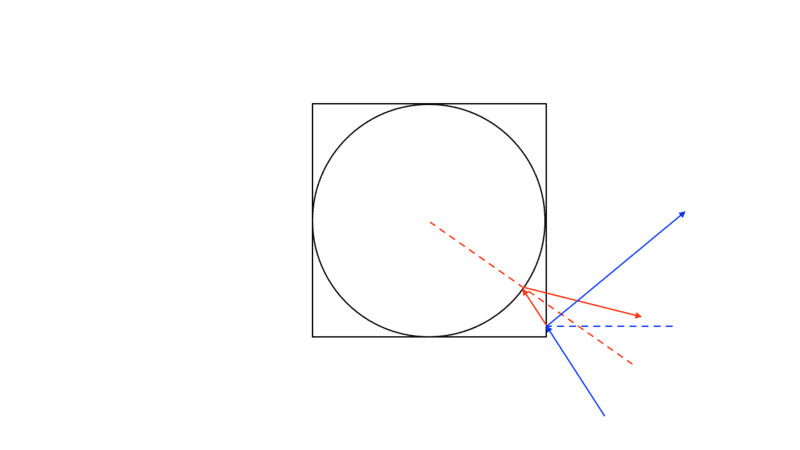The red one is the trajectory according to the round post, the blue one is according to the square
Here we can see that the blue path will go in but the red won't.

kshitij
So, the sketch should look something like this?
View attachment 282688
The red one is the trajectory according to the round post, the blue one is according to the square
Here we can see that the blue path will go in but the red won't.
But there are other possibilities like this one below,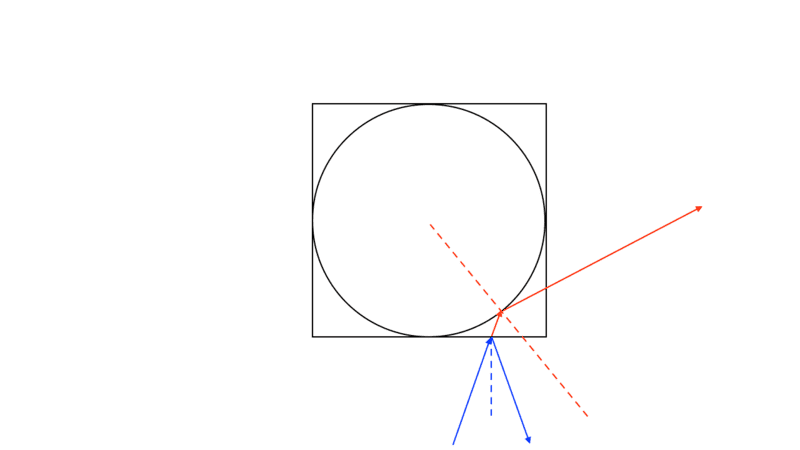Here we can clearly see that the blue path (square post) won't go in but the red (round) will go in.

kshitij
We would say that this is an (exact) solution to a simplified problem, rather than a 'rough idea' of the solution of the main problem.
If that is the case then I'm only interested in finding the solution to this simplified problem, forget about the real life problem.

Last edited:
kshitij
Suppose the ball approaches at an angle θ to the normal (so an angle of zero is normal to the goal line) and at a lateral offset from the centre of the post of x.
So we only need to consider x in the range -R to +R, the positive side being where the actual goal is.
E.g. with θ=0, it will go in the goal if x>R/2, yes?
Can you generalise that?
Again, I would need a sketch to understand what do you mean here?
is it like this?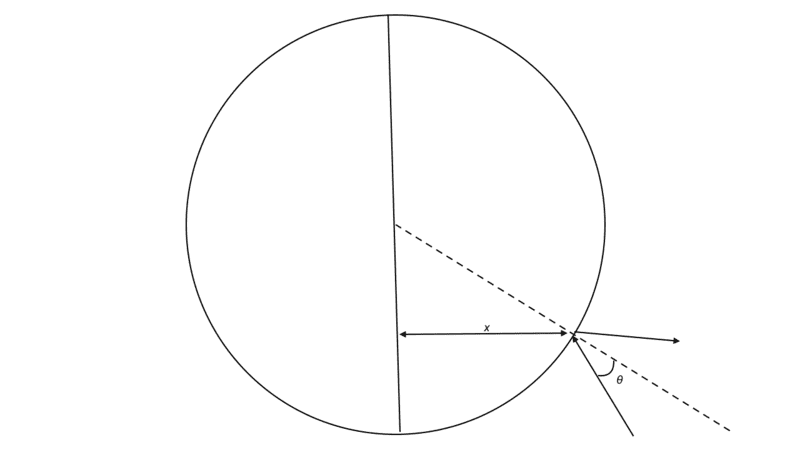If yes, then according to your example I cannot see how the ball will go in (if the ball hits the post legally) for θ=0 case.

kshitij
Suppose the ball approaches at an angle θ to the normal (so an agle of zero is normal to the goal line) and at a lateral offset from the centre of the post of x.
So we only need to consider x in the range -R to +R, the positive side being where the actual goal is.
E.g. with θ=0, it will go in the goal if x>R/2, yes?
Can you generalise that?
On re-reading this, I think this sketch (below) makes more sense,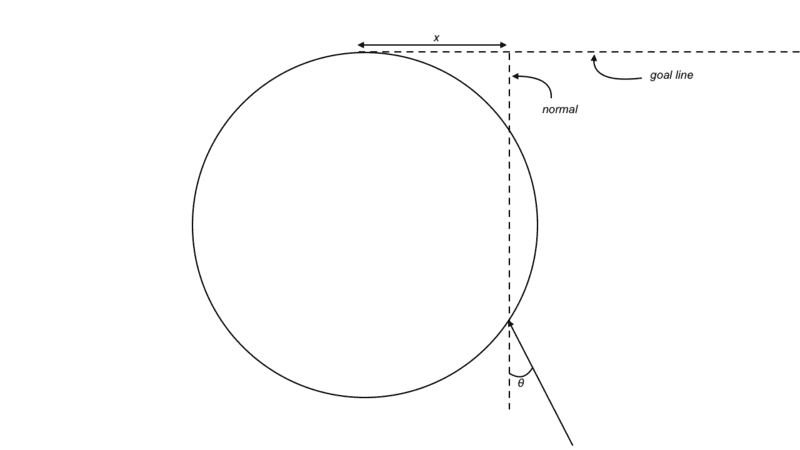kshitij
On re-reading this, I think this sketch (below) makes more sense,
View attachment 282694
But again, this figure still can't explain how can you say this,
E.g. with θ=0, it will go in the goal if x>R/2, yes?

Homework Helper
2022 Award
Everyone understands that this is a straightforward calculation of the 2D differential scattering cross-section for a few different potentials?
No need to re-invent the wheel here.

••pbuk and kshitij
kshitij
Also in future if anyone wants to say something like, the ball strikes the inside/pitch-side quadrant of a round post, inside surface of a square post, etc please use the reference figures as shown below,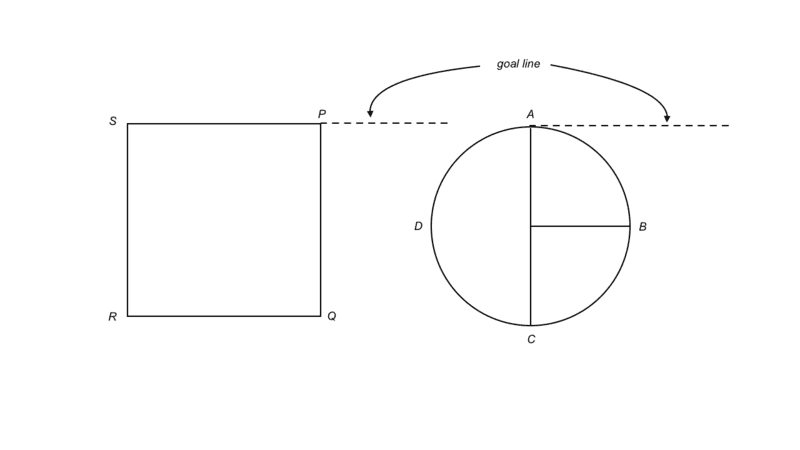kshitij
Everyone understands that this is a straightforward calculation of the 2D differential scattering cross-section for a few different potentials?
No need to re-invent the wheel here.
The what?

Homework Helper
Gold Member
2022 Award
On re-reading this, I think this sketch (below) makes more sense,
View attachment 282694
Yes, that's the picture I have in mind.
If you set theta to zero and increase x a bit (it is less than R/sqrt(2) in your example) it will make a glancing blow on the post and continue towards the goal line. It will be a goal if the other post is sufficiently far away.

kshitij
Yes, that's the picture I have in mind.
If you set theta to zero and increase x a bit (it is less than R/sqrt(2) in your example) it will make a glancing blow on the post and continue towards the goal line. It will be a goal if the other post is sufficiently far away.
I'll have to disagree on that one, I think the limiting case should look like this,
Homework Statement:: Consider two shapes of goal posts, one with a circular cross-section and the other with square. Which of these posts have a greater probability of a shot hitting the post, go in?
Relevant Equations:: Laws of reflection

Limiting/critical incidence,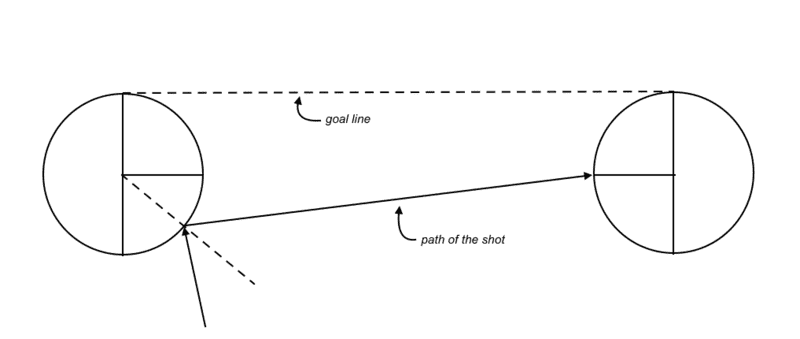Homework Statement:: Consider two shapes of goal posts, one with a circular cross-section and the other with square. Which of these posts have a greater probability of a shot hitting the post, go in?
Relevant Equations:: Laws of reflection

The critical value of angle of incidence happens when the reflected ray from the sector BC of one post hits the sector AB of the other post.

Staff Emeritus
Homework Helper
Gold Member
As I mentioned in my post a square post has 33% chance of shot going in after hitting the post
Which, as has been pointed out, is wrong. You cannot just state this without justification. A ball, depending on the angle, will not be equally likely to hit each side of the post.

kshitij
A ball, depending on the angle, will not be equally likely to hit each side of the post.
I said that the ball is equally likely to come from any angle

kshitij
Which, as has been pointed out, is wrong. You cannot just state this without justification. A ball, depending on the angle, will not be equally likely to hit each side of the post.
I did realize that my original question isn't realistically answerable because there are lots of factors to consider.

That's why right now I'm focusing on the modified version of my question.

Homework Helper
Gold Member
2022 Award
I'll have to disagree on that one, I think the limiting case should look like this,
I said sufficiently far away. Make the other post further away and it goes in.
But even in your diagram, that is not quite the limiting case. It can hit the far post just below the mid point, then bounce back to the first post, just a little closer to the midpoint, then... you get the idea.

kshitij
Make the other post further away and it goes in.
But the distances between the posts are fixed and finite.
But even in your diagram, that is not quite the limiting case. It can hit the far post just below the mid point, then bounce back to the first post, just a little closer to the midpoint, then... you get the idea.
If that's the case then it should eventually go in no matter where the ball strikes on sector BC? because it will keep bouncing between the posts till it gets closer to the midpoint of any post??

kshitij
If that's the case then it should eventually go in no matter where the ball strikes on sector BC? because it will keep bouncing between the posts till it gets closer to the midpoint of any post??
I don't think it works this way (see below, the blue lines are the path of the ball)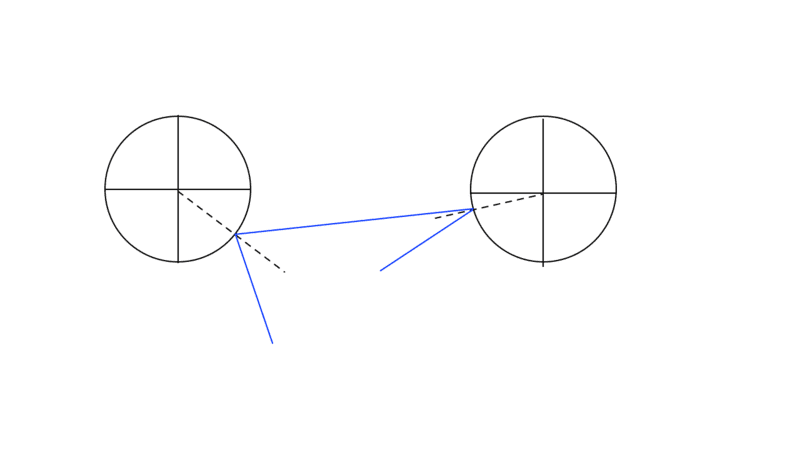As you can see any ball striking below the midpoint of the far post, will never go in (as I suspected in the beginning)

Homework Helper
Gold Member
But there are other possibilities like this one below,
View attachment 282689
Good point. Here we can clearly see that the blue path (square post) won't go in but the red (round) will go in. In fact there are some trajectories of this kind where a ball (even a full size ball) will enter the goal without contacting a round post that would collide with (the corner of) a square post.

This offsets the advantage of a square post for a shot from between the posts: whether it eliminates or even reverses that advantage depends on what weighting you place on each trajectory.

kshitij
Homework Statement:: Consider two shapes of goal posts, one with a circular cross-section and the other with square. Which of these posts have a greater probability of a shot hitting the post, go in?
Relevant Equations:: Laws of reflection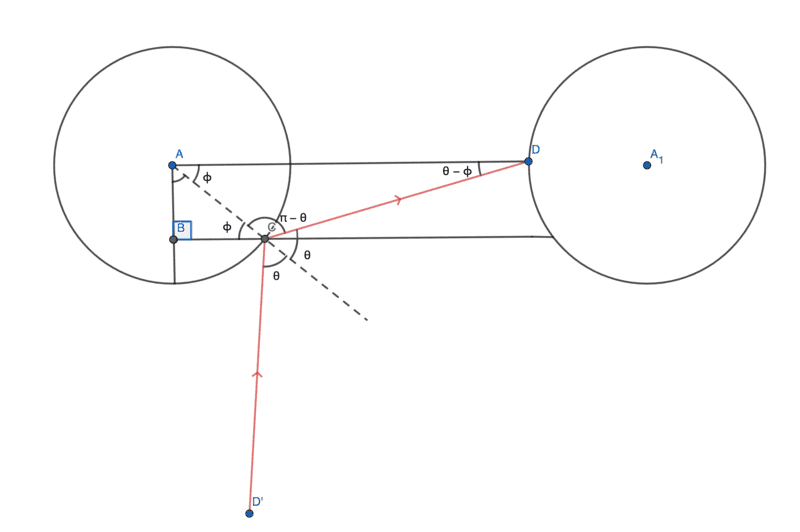Thus, I believe that this is the correct limiting case.

Homework Helper
Gold Member
Everyone understands that this is a straightforward calculation of the 2D differential scattering cross-section for a few different potentials?
Yes, I have been trying to find a relevant paper but I can't.

•hutchphd
kshitij
what weighting you place on each trajectory.
What do you mean by this? Does it mean something like how probable the ball is to come from any given angle? If so then I had assumed that it is equally likely to come from any angle

Homework Helper
Gold Member
What do you mean by this? Does it mean something like how probable the ball is to come from any given angle? If so then I had assumed that it is equally likely to come from any angle
Not just angle, it makes a difference whether the ball is heading for the front corner of the post or the middle, or somewhere in between.

So now you have two assumptions on which you are basing your calculations:
• The ball is of negligible size compared to the post (whereas in fact the ball is larger than the post)
• Shots from e.g. 10cm from the corner flag are equally as likely as shots from in front of the goal (which does not match my (very limited) observation of real play)

Are you still going to be happy with your conclusions?

•berkeman
kshitij
• Shots from e.g. 10cm from the corner flag are equally as likely as shots from in front of the goal (which does not match my (very limited) observation of real play)
I'm happy with this particular assumption because the play is randomly distributed around each post, that is, its allowed for any player to take a shot from anywhere on the field, so given enough time, yes I think that shots from the corner flag are equally as likely as shots from the front of the goal.

However there isn't anything I could do about the other assumption, I'll have to assume the ball to be a point mass, otherwise its impossible to calculate anything. But as mentioned earlier, I would still be happy to find out results about the point mass.

Homework Helper
Thus, I believe that this is the correct limiting case.
No. This is not the correct limiting case.Here you have the ball striking the left hand goal post somewhere near the 45 degree line and rebounding into the right hand goal post exactly at it's 9 o'clock position. The ball will then continue, rebounding leftward at the same angle (##\Theta - \Phi##) above the horizontal as it arrived from below. That rebound angle is clearly more than good enough to score. You need a rebound angle that is only exactly good enough to score.

kshitij
No. This is not the correct limiting case.
View attachment 282714
Here you have the ball striking the left hand goal post somewhere near the 45 degree line and rebounding into the right hand goal post exactly at it's 9 o'clock position. The ball will then continue, rebounding leftward at the same angle (##\Theta - \Phi##) above the horizontal as it arrived from below. That rebound angle is clearly more than good enough to score. You need a rebound angle that is only exactly good enough to score.
That is the limiting case of ##\theta## for any fixed value of ##\phi##

Homework Helper
That is the limiting case of ##\theta## for any fixed value of ##\phi##
How so? Smaller angles of theta can result in strikes lower on the right hand goal post that still go in.

kshitij
How so? Smaller angles of theta can result in strikes lower on the right hand goal post that still go in.
For smaller angles of ##\theta## the ball would strike below the point D so how can the ball still go in?

Homework Helper
Gold Member
2022 Award
For smaller angles of ##\theta## the ball would strike below the point D so how can the ball still go in?
It is easiest to see it by running it in reverse.
Suppose the ball eventually hits the half way around point of one of the posts, but does so approaching from almost parallel to the goal line, making some tiny angle ##\alpha## to it.
Trace that back to its last bounce off the other post, and back again towards the first, and so on until it misses a post. Clearly, by making ##\alpha## small enough there is no limit to the number of bounces.
The algebra will be messy...
Edit: it goes like this. I'll describe it as though the ball starts at the halfway around point of one post and progressively bounces out of the goal.
Let the posts be 1, 2 and the distance between them along their line of centres, L, be D>>R.
From 1, it hits 2 roughly ##D\alpha## from L, at a point subtending angle ##\frac DR\alpha## to that line at the centre of 2.
It bounces from there at an angle ##(1+2\frac DR)\alpha## to L, do you see that?
Can you generalise it to n bounces?

Last edited:
Homework Helper
Gold Member
2022 Award
What do you mean by this? Does it mean something like how probable the ball is to come from any given angle? If so then I had assumed that it is equally likely to come from any angle

Yes, you can choose it to be equally likely from any angle within the pitch, and for a given angle equally likely at any lateral displacement from the line that would have it hitting the post along the normal to it (of those displacements for which it would make contact). That is what I did for my result in post #28.

But you could instead say, e.g., it is equally likely from anywhere within the pitch, and at any angle from that point that touches the post. This gives a different distribution since a shot from a wide angle is relatively unlikely.

In reality, shots that touch the inner part of the post are more likely than shots that touch the outer part because the player is attempting to get it in the goal.

kshitij
It is easiest to see it by running it in reverse.
Suppose the ball eventually hits the half way around point of one of the posts, but does so approaching from almost parallel to the goal line, making some tiny angle ##\alpha## to it.
Trace that back to its last bounce off the other post, and back again towards the first, and so on until it misses a post. Clearly, by making ##\alpha## small enough there is no limit to the number of bounces.
The algebra will be messy.
I'll need a figure to understand that but right now I have to go, maybe I'll come back to that later.